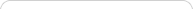##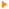Teaching with Sketchpad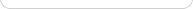•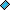- plays a video
•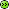- opens a web page# Geometry

##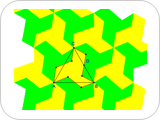Tessellations That Use Rotations

Students construct an irregularly-shaped tile based on an equilateral triangle, and then use rotation to tessellate the plane with it.

##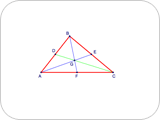Medians in a Triangle

Students construct a triangle and its medians. They observe the concurrence of the medians, measure distances to observe how the centroid divides each median, and make a custom tool for constructing the centroid of a given triangle.

##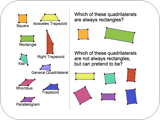Quadrilateral Pretenders: Classifying Quadrilaterals

Students drag edges and vertices of various Sketchpad quadrilaterals to discover which are constructed to have specific characteristics. As they make distinctions on the basis of characteristics, they deepen their understanding of the definitions of various quadrilaterals, their properties, and the relationships among them.

##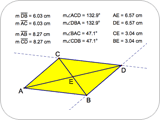Meet the Parallelogram: Properties of Parallelograms

Students construct a parallelogram, measure side lengths and angles, and observe that opposite sides are congruent, opposite angles are congruent, and consecutive angles are supplementary. Then they construct the diagonals, measure the distances from the vertices to the point of intersection, and discover that the diagonals bisect each other.

##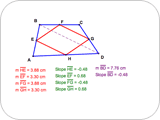Midpoint Quadrilaterals

Students connect the midpoints of a quadrilateral to construct a midpoint quadrilateral. They discover that the midpoint quadrilateral is a parallelogram and prove this conjecture.

##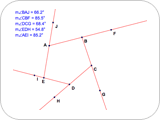Exterior Angles in a Polygon

Students construct a convex polygon and make a conjecture about the sum of the measures of its exterior angles. They dilate the polygon to approximately a single point to create a visual proof by dilation that the sum of the measures of the exterior angles of a convex polygon is what they conjectured.

##Chords in a Circle

Students explore the properties of chords in a circle. They construct a chord and its perpendicular bisector and discover a relationship between the chord’s length and its distance to the center of the circle, and they investigate and write a conjecture about congruent chords in a circle.

##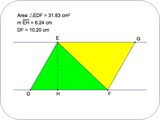Parallel Pairs: Parallelogram and Triangle Area

Students explore the relationship between the areas of parallelograms and triangles using a process called shearing. Students discover that shearing does not affect the area, but changing the lengths of the height and base does. Based on their observations, students write formulas for area of a parallelogram and area of a triangle.

##Pyramid Dissection: Surface Area

Students find the surface area of a regular pyramid (a pyramid with a regular polygon base) using a net that appears along a three-dimensional view of the pyramid. They ensure the generality of their results by changing the dimensions and number of faces of the base. By increasing the number of faces, students extend their results to the surface area of a cone, giving them an informal opportunity to think about limits.

##Visual Demonstration of the Pythagorean Theorem

Students investigate a visual demonstration of the Pythagorean theorem based on Euclid’s proof. They use shearing to modify the squares on the sides of a right triangle to create congruent shapes without changing the areas of the original squares, and then explain why these shapes demonstrate the Pythagorean theorem.